# ACT Mathematics: Trignometry Quiz

This is an MCQ-based quiz on the topic of the ACT Mathematics Trignometry Quiz.

This set of MCQs helps you brush up on the important mathematics topic and prepare you to dive into skill practice and expand your knowledge to tackle the question and answer it carefully.

Start Quiz

For the triangles in the figure given, which of the following is closest to the length of line NO?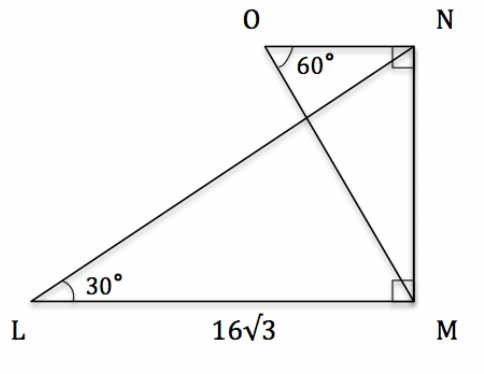7

6

9

10

For the above triangle, o=14 and a=28. Find θ.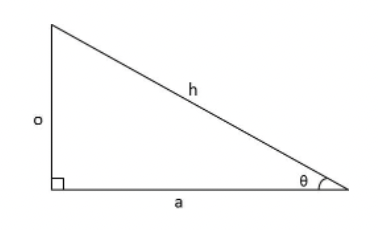27

30

35

20

In the above triangle, o=4 and a=7. Find θ.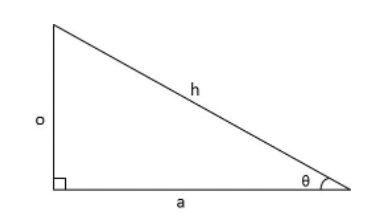40

35

25

30

For the above triangle, o=21 and a=8. Find θ.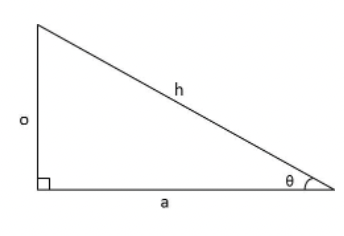70

75

89

15

If the sine of an angle equals 2/3, and the cosine of the same angle equals 5/12, what is the tangent of the angle?

8/5

4/5

6

7/5

Triangle ABC shown is a right triangle. If the tangent of angle C is 37, what is the length of segment BC?6

7

8

9

Consider a right triangle with an inner angle x(x<90∘).

If

cosx=35

and

sinx=45

what is tanx?

5

1/5

4/3

1

What is the tangent of C in the given right triangle??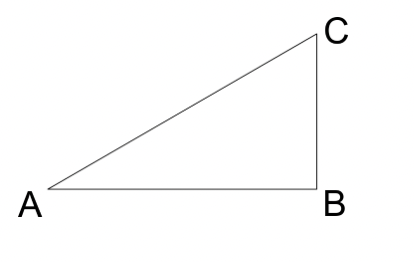AB/BC

AB/AC

CB/AB

CB/AC

Quiz/Test Summary
Title: ACT Mathematics: Trignometry Quiz
Questions: 8
Contributed by: